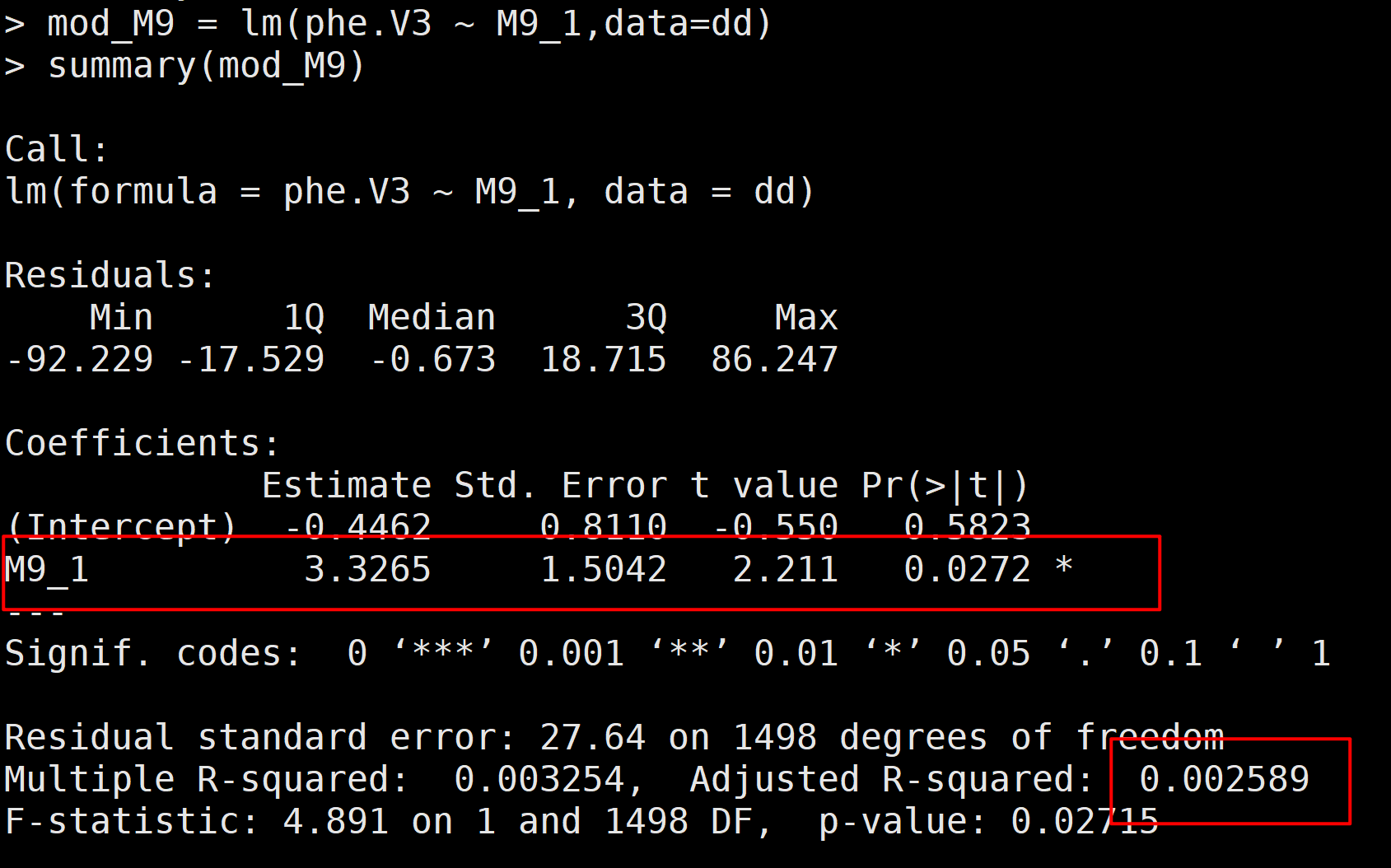# GWAS分析中：为何有些SNP效应值大却不显著？

## 1. 错误的理解

「事实上：」效应值和显著性是两码事！

## 2. 用代码说话

GWAS分析中，最简单的就是一般线性模型（GLM），而GLM模型进行的GWAS分析中，用R语言实现的代码如下：

mod_M7 = lm(phe.V3 ~ M7_1,data=dd)summary(mod_M7)

「结果：」这个里面：

• Estimate就是效应值：3.3265，这个就是SNP M9的效应值
• Pr就是P值：0.0272，这个就是SNP M9的P值

## 3. GWAS分析的思路

• 1，将SNP的分型转化为0, 1, 2的形式，主效纯合（major）编码为0，杂合编码为1，次等位纯合编码为2
• 2，x变量为0,1,2的数值，y变量为性状表型值
• 3，对x和y做回归分析y ~ a*x + b，a为效应值，a是否显著的P值，为SNP的P值

## 4. SNP效应值很大，却不显著

# SNP 分型set.seed(666)x = rep(c(0,1,2),3)x# 模拟表型值y = 12*x + rnorm(9)*10# 作回归分析mod = lm(y ~ x)summary(mod)# 作图dd = data.frame(x,y)ddggplot(dd,aes(x=x,y=y)) + geom_point() + stat_smooth(method='lm',formula = y~x,colour='red')

> dd  x          y1 0   7.5331102 1  32.1435473 2  20.4486554 0  20.2816785 1 -10.1687456 2  31.5839627 0 -13.0618538 1   3.9748049 2   6.077592

> # 平均值> aggregate(y~x,data = dd,mean)  x         y1 0  4.9176452 1  8.6498693 2 19.370070

「看一下回归分析的结果：」

> # 作回归分析> mod = lm(y ~ x)> summary(mod)Call:lm(formula = y ~ x)Residuals:    Min      1Q  Median      3Q     Max -21.148 -12.128   2.243  13.379  21.164 Coefficients:            Estimate Std. Error t value Pr(>|t|)(Intercept)    3.753      8.558   0.439    0.674x              7.226      6.629   1.090    0.312Residual standard error: 16.24 on 7 degrees of freedomMultiple R-squared:  0.1451, Adjusted R-squared:  0.023 F-statistic: 1.188 on 1 and 7 DF,  p-value: 0.3118

「为何会出现这种情况呢？」我们画一个散点图看一下分布情况：

library(ggplot2)ggplot(dd,aes(x=x,y=y)) + geom_point() + stat_smooth(method='lm',formula = y~x,colour='red')可以看到，分型为1的表型值，有一个非常高，达到了31，相当于在进行T检验时，标准误se比较高，导致P值较大，不显著。

## 5. SNP效应值很小，却极显著

# SNP 分型set.seed(666)x = rep(c(0,1,2),3)x# 模拟表型值y = 0.1*x + rnorm(9)*0.01# 作回归分析mod = lm(y ~ x)summary(mod)# 作图dd = data.frame(x,y)ddlibrary(ggplot2)ggplot(dd,aes(x=x,y=y)) + geom_point() + stat_smooth(method='lm',formula = y~x,colour='red')# 平均值aggregate(y~x,data = dd,mean)

「模拟的数据如下：」

> dd  x           y1 0  0.007533112 1  0.120143553 2  0.196448664 0  0.020281685 1  0.077831266 2  0.207583967 0 -0.013061858 1  0.091974809 2  0.18207759

> aggregate(y~x,data = dd,mean)  x           y1 0 0.0049176452 1 0.0966498693 2 0.195370070

「回归分析结果：」

> # 作回归分析> mod = lm(y ~ x)> summary(mod)Call:lm(formula = y ~ x)Residuals:      Min        1Q    Median        3Q       Max -0.021148 -0.012128  0.002243  0.013379  0.021164 Coefficients:            Estimate Std. Error t value Pr(>|t|)    (Intercept) 0.003753   0.008558   0.439    0.674    x           0.095226   0.006629  14.365 1.89e-06 ***---Signif. codes:  0 ‘***’ 0.001 ‘**’ 0.01 ‘*’ 0.05 ‘.’ 0.1 ‘ ’ 1Residual standard error: 0.01624 on 7 degrees of freedomMultiple R-squared:  0.9672, Adjusted R-squared:  0.9625 F-statistic: 206.4 on 1 and 7 DF,  p-value: 1.886e-06

「作图看一下：」虽然回归系数（SNP效应值很小），但是每个分型对应的表型值相差不多，组内变异（同一种基因型的表型值）远远小于组间变异（不同SNP分型对应的表型值），导致进行T检验时，达到极显著水平。

## 6. 从生物学水平如何解释？

「SNP效应大但不显著的位点：」有可能SNP是有大效应的，但是容易受到环境影响，或者由于群体的结构（某个群体内聚集），或者由于某些异常值导致这种情况。也有可能本身就是不显著的。

「林子大了，什么鸟都有！」毕竟SNP都是上万，上十万，上百万的量。而表型值都是上百，上千，很少达到上万的，这就存在很多种情况，导致有些SNP效应值很大，但是不显著，有些SNP效应值很小，但是极显著。这里需要区分对待一下。

## 7. GWAS系列相关

http://wap.sciencenet.cn/blog-2577109-1250860.html

## 全部精选博文导读

GMT+8, 2021-11-30 03:11